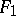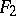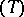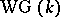# Witt theorem

(diff) ← Older revision | Latest revision (diff) | Newer revision → (diff)
Any isometry between two subspacesandof a finite-dimensional vector space, defined over a fieldof characteristic different from 2 and provided with a metric structure induced from a non-degenerate symmetric or skew-symmetric bilinear form, may be extended to a metric automorphism of the entire space. The theorem was first obtained by E. Witt .
Witt's theorem may also be proved under wider assumptions onand, . In fact, the theorem remains valid ifis a skew-field,is a finite-dimensional left-module andis a non-degenerate-Hermitian form (with respect to some fixed involutory anti-automorphismof, cf. Hermitian form) satisfying the following condition: For anythere exists an elementsuch that(property). Propertyholds if, for example,is a Hermitian form and the characteristic ofis different from 2, or ifis an alternating form. Witt's theorem is also valid ifis a field andis the symmetric bilinear form associated with a non-degenerate quadratic formon. It follows from Witt's theorem that the group of metric automorphisms oftransitively permutes the totally-isotropic subspaces of the same dimension and that all maximal totally-isotropic subspaces inhave the same dimension (the Witt index of). A second consequence of Witt's theorem may be stated as follows: The isometry classes of non-degenerate symmetric bilinear forms of finite rank overwith direct orthogonal sum form a monoid with cancellation; the canonical mapping of this monoid into its Grothendieck group is injective. The groupis called the Witt–Grothendieck groupof; the tensor product of forms induces on it the structure of a ring, which is known as the Witt–Grothendieck of.